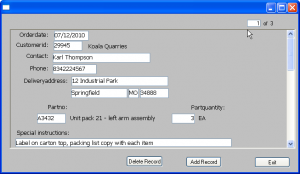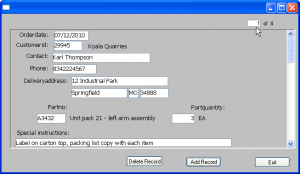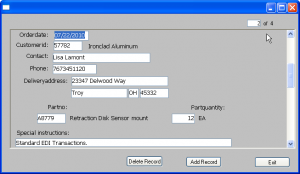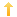## PowerBuilder – Item counter for datawindow entries

Posted on Wednesday, October 20th, 2010 at 8:20 pm in

This is a PFC based component I build for an application used to create purchasing requisitions. The main datawindow was used to enter new ‘documents’ and was too large to allow for more than one row to be visible at a time. Rather than use a common ‘vcr’ type control I created one which shows the current row number, the total number of rows, and the ability to go to any row within the total.

The component, u_itemcounter, is inherited from u_dw.

The dataobject, d_itemcounter, has an external datasource with three columns:

```table(column=(type=number updatewhereclause=yes name=itmcount dbname="itmcount" initial="0" )
column=(type=char(2) updatewhereclause=yes name=of dbname="of" initial="of" )
column=(type=number updatewhereclause=yes name=itmtotal dbname="itmtotal" initial="0" )
)
```

Instance variables:

```u_dw	idw_source
long	il_currentrow
long	il_rowcount```

The code in the control is as follows:

```public function integer of_setsource (ref u_dw adw_source);
// sets the source datawindow
integer li_return
li_return = 0
RETURN li_return
end function

public subroutine of_setmaxrow (long al_row);
il_rowcount = al_row
this.setitem(1,'itmtotal',al_row)
end subroutine

public function long of_getcurrentrow ();
RETURN il_currentrow
end function

public subroutine of_setcurrentrow (long al_row);
il_currentrow = al_row
this.setitem(1,'itmcount',al_row)
end subroutine

event constructor;call super::constructor;
this.of_setbase(TRUE)
this.of_SetRowSelect(FALSE)
this.of_SetRowManager(FALSE)
this.of_SetSort(FALSE)
this.setrowfocusindicator(Off!)
end event

event losefocus;call super::losefocus;
long ll_data
IF this.rowcount( ) < 1 THEN RETURN
ll_data = this.getitemnumber(1,'itmcount')
IF ll_data > il_rowcount THEN
ll_data = il_rowcount
END IF
of_setcurrentrow (ll_data)
idw_source.setrow(il_currentrow)
idw_source.scrolltorow(il_currentrow)
end event

event itemchanged;call super::itemchanged;
IF long(data) > il_rowcount THEN
// ERROR CONDITION
RETURN 1
ELSE
of_setcurrentrow (long(data))
idw_source.setrow(row)
idw_source.scrolltorow(row)
END IF
end event

event type long pfc_insertrow();
call super::pfc_insertrow;
long	ll_maxrow
ll_maxrow = idw_source.rowcount()
of_setmaxrow(ll_maxrow)
of_setcurrentrow(ANCESTORRETURNVALUE)
RETURN ANCESTORRETURNVALUE
end event```

To implement the control, place it on your window in a suitable location near the ‘master’ datawindow. In my case just above the master datawindow on the left edge. I named the control ‘dw_counter.’

When window opens, master control has three recordsIn the constructor event of the master datawindow add the following:

```u_dw ldw
ldw = THIS
dw_counter.of_setsource(ldw) // set the link to the counter object```

```long ll_rc
ll_rc = this.rowcount()
IF (dw_counter.rowcount() < 1) THEN
dw_counter.event trigger pfc_insertrow()
ELSE
dw_counter.of_setmaxrow(ll_rc)
END IF
RETURN ANCESTORRETURNVALUE```

In the pfc_predeleterow method the following:

```long ll_row
// reset the counter dw
IF ANCESTORRETURNVALUE = CONTINUE_ACTION THEN
ll_row = dw_counter.of_getcurrentrow()
IF (ll_row = this.Rowcount()) THEN
dw_counter.of_setcurrentrow(ll_row - 1)
dw_counter.of_setmaxrow(ll_row - 1)
ELSE
dw_counter.of_setmaxrow(this.Rowcount() - 1 )
END IF
END IF
RETURN ANCESTORRETURNVALUE```

And finally in the scrollvertical event the following:

```long ll_row
string ls_row
// set counter to current row when scrolling
ls_row = this.Object.Datawindow.FirstRowOnPage
ll_row = Long(ls_row)
dw_counter.of_setcurrentrow(ll_row)```

The master datawindow in the example window contains three records. To set the counter properly when the window opens the following is put in the pfc_postopen event:

`dw_counter.event pfc_insertrow() // makes the control visible`Moved to record two in the master datawindowIf you choose to create your component without the PFC you will obviously have to change the code.